Next: Some Practical Considerations Up: Optimal Extraction Previous: General Principles

### 7.10.2 Mathematical Details

At a givenon order m, there is a true signal level equivalent to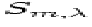photons accumulated over n exposures that were added together. This signal is spread over a vertical extent of approximately 20 pixels. The actual signal sensed in each pixel is quantized and has the usual Poisson statistical fluctuations. On average (in the absence of statistical uncertainties) the expected numerical outcome in each pixel identified by a subscript i is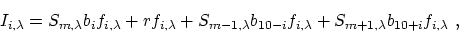(6)
where
i
is the index that describes the y location of a pixel or horizontal slice, with i=0 being set at the location of maximum intensity for order m.
bi
is the fraction of energy deposited in a particular horizontal section i of an order (normalized such that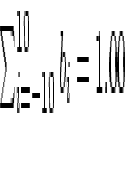).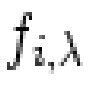is the sensitivity pattern's attenuation function averaged over n images. It is normalized so that an interblob region has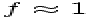, and thuswould be the signal registered if there were no blobs with reduced sensitivity.
r
is the background illumination level.

 Figure 12: An illustration of how to determine the most likely spectrum intensity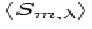along an order m at wavelength. The height of the surface above the horizontal x-i plane represents the recorded image intensity. Small squares show measurements of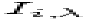from i=-5 to +5. The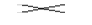's show the samples taken to determine the most likely intensities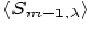and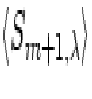of the contaminating orders. The entire pattern is elevated above a general background r, and the holes represent depressions in the sensitivity.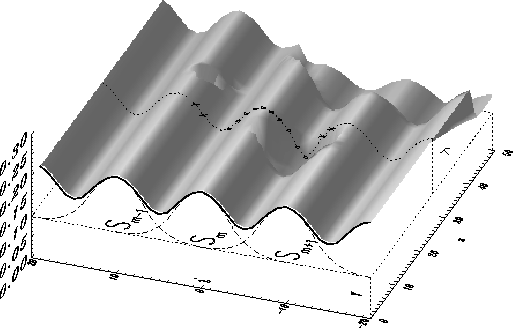The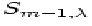and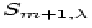terms are signals in the adjacent orders that can contaminate our sampling of. They must be evaluated and subtracted out, and because of their uncertainties, they lower the reliability of outlying samples of-- an effect that must be recognized when the relative weights are assigned for different i. To estimate these contamination contributions, we sampled the adjacent orders at positions near their cores, but slightly offset in the direction of the order m (see Fig. 12). This offset insures, to first order, that small errors in centering on order m automatically adjust the contamination correction in the right direction.

In setting up formulae for the variances of quantities that would come from hypothetical, repeated trials of the experiment, we must express the outcome using a general value for S, called Sm as distinguished from, because we do not want the weight factors to be influenced by the local chance fluctuations on top of the desired and contamination signals. In practice, Sm can be pictured in terms of an average ofover a range ofthat is large enough to make such fluctuations inconsequential.

Imagine that we could perform repeated trial measurements of Ii. We should expect to find a variance,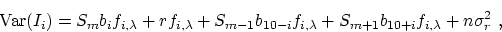(7)
whereis the rms readout plus CCD dark current noise (§) (expressed as an amplitude relative to that of a single photoevent). Numerically,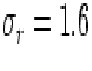for a 511-frame integration over 34 s. The most effective approach for reconstructing S is to evaluate at allthe individual estimates for the most probable values for, which we designate as: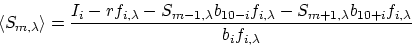(8)
In the numerator, the first term is the basic measurement that has random fluctuations governed by Eq. 7. The second term is an ultraviolet background correction term that does not vary (i.e., it is a global correction, except for a general trend that follows the blaze function of the echelle grating). The third and fourth terms are correction factors that must be applied to cancel out the contamination signals from adjacent orders. These two terms have variations of their own that corrupt the correction process, since we can not measureandwith perfect accuracy. The magnitude of these corrections depend on exactly how we sample the adjacent orders (preferably, near enough to their centers that we do not have to worry about these orders being contaminated!)

Associated withis its variance,

whereand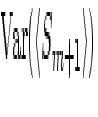are determined through Eq. 7 without the correction terms because of the deliberate, very limited sampling reasonably near these orders' centers (to avoid the complexity of second order contamination corrections upon the first order ones).

Specifically, the intensities of the adjacent orders are determined by two measurements in each case, such that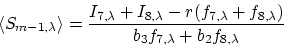(9)
and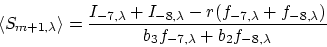(10)
The choice of using i = 7 and 8 is a matter of judgement. Measurements closer to the centers of the other orders will be more accurate. However, we then lose the automatic compensation for centering errors. The simple sum without weight factors reduces the complexity of the equation and is justified on the basis that b2 is not very different from b3. As before, the expected variance in the estimate is given by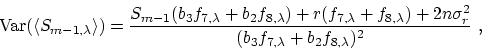(11)
and likewise for.

Now that we have derived formulae for the best estimate ofand its reliability for a given value of i, we must combine the measurements at different i in an optimum manner. We also need to have a measure of the uncertainty in the outcome, in case we wish to combine the extraction with other ones.

For measurements with different uncertainties, the standard way to combine them is by evaluating a weighted average, with weights that are inversely proportional to the variances: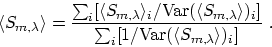(12)
The error in the result is given by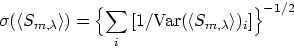(13)
The combination shown in Eq. 13 is not strictly ideal, because the correction terms for interference from adjacent orders will have correlated errors. This effect is probably rather small, since errors are strongly dominated by the background fluctuations (in the r terms) in cases where the correction amounts to much.Next: Some Practical Considerations Up: Optimal Extraction Previous: General Principles
Karen Levay
12/15/1998